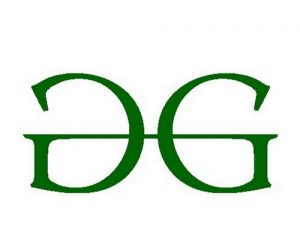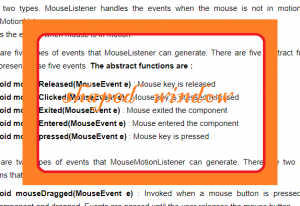GFG App
Open AppBrowser
Continue

# JSwing | Create Translucent and Shaped Windows in Java

Per – Pixel Translucency allows the programmer to control the translucency of a specific pixel over others. we will use this property to create a shaped window.
In this article we will try to implement shaped window in java by creating a transparent window and then painting the panel and then adding the Panel to the window.

• setBackground(Color c)method is used to set the background color to color c.
• color class is used to create a color of required transparency.

Methods :

1. setBackground(Color c) : method to set the background color to color c
2. color(int r, int g, int b, int alpha) : creates a new color with specified red, green, blue and alpha value. where alpha is the value of translucency where 255 is opaque and 0 is transparent .
3. getRGB(int x, int y) : returns the RGB value of the Coordinate x, y
4. setColor(Color c) : set the color of graphics to c.

Examples:

1. Example 1

Input :Output:2. Example 2

Input :Output:3. Example 3 :
Input :Output 3 :Explanation : Here, in the input we can see that the background is made transparent whereas the
symbol of GeeksforGeeks remains as it was. Through the transparent background we can see the window below. We have separated the logo from its background and painted it on a transparent window .
The program below will illustrate how to create a shaped window using per – pixel translucency.

## Java

 `// Java Program to implement the shaped window ` `import` `javax.swing.*; ` `import` `java.awt.*; ` `import` `java.awt.image.*; ` `import` `java.io.*; ` `import` `javax.imageio.*; ` `class` `solve ``extends` `JFrame { ` ` `  `    ``// main class ` `    ``public` `static` `void` `main(String[] args) ` `    ``{ ` `        ``// try block ` `        ``try` `{ ` ` `  `            ``// create a window ` `            ``JWindow w = ``new` `JWindow(); ` ` `  `            ``// set a transparent  background of the window ` `            ``w.setBackground(``new` `Color(``0``, ``0``, ``0``, ``0``)); ` ` `  `            ``// read the image ` `            ``BufferedImage i = ImageIO.read(``new` `File(``"f:/gfg.png"``)); ` ` `  `            ``// create a panel ` `            ``JPanel p = ``new` `JPanel() { ` ` `  `                ``// paint the panel ` `                ``public` `void` `paintComponent(Graphics g) ` `                ``{ ` `                    ``// extract the pixel of the image ` `                    ``for` `(``int` `ii = ``1``; ii < i.getHeight(); ii++) ` `                        ``for` `(``int` `j = ``1``; j < i.getWidth(); j++) { ` `                            ``// get the color of pixel ` `                            ``Color ty = ``new` `Color(i.getRGB(j, ii)); ` ` `  `                            ``// if the color is more than 78 % white ignore it keep it transparent ` `                            ``if` `(ty.getRed() > ``200` `&& ty.getGreen() > ``200` `&& ty.getBlue() > ``200``) ` `                                ``g.setColor(``new` `Color(``0``, ``0``, ``0``, ``0``)); ` `                            ``// else set  the color ` `                            ``else` `                                ``g.setColor(``new` `Color(i.getRGB(j, ii))); ` ` `  `                            ``// draw a pixel using a line. ` `                            ``g.drawLine(j, ii, j, ii); ` `                        ``} ` `                ``} ` `            ``}; ` ` `  `            ``// add panel ` `            ``w.add(p); ` ` `  `            ``// set the location ` `            ``w.setLocation(``350``, ``300``); ` ` `  `            ``// set the size of the window ` `            ``w.setSize(``900``, ``900``); ` ` `  `            ``// set the visibility of the window ` `            ``w.setVisible(``true``); ` `        ``} ` `        ``// catch any exception ` `        ``catch` `(Exception e) { ` ` `  `            ``// show the error ` `            ``System.err.println(e.getMessage()); ` `        ``} ` `    ``} ` `} `

Input 1 :Output 1 :Input 2 :Output 2 :Input 3 :Output 3 :Note: I have chosen the color white as the background so I have separated it from the image and made it transparent. Its upon the discretion of the programmer to choose the background of the image .

Note : The above programs might not run in an online compiler please use an offline IDE.
It is recommended to use latest version of java to run the above programs, user might face problems if older versions of java are used.

My Personal Notes arrow_drop_up